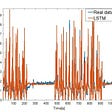# Neural Network for predictions. How to improve it

Hi everyone,

I am trying to build a code with the NN toolbox, able to predict at time t+2 the Manganese content in a reservoir, given as inputs other 7 variables (among these, also the Mn at time t=0).

While I am waiting for far more data, which definitely will improve the performance, I am trying to play with the several options through the network to improve it. You find the code below:

`_  inputs = Inputs7old';  targets = Mnt2old';% Create a Fitting NetworkhiddenLayerSize = 10;FIT1 = fitnet(hiddenLayerSize);FIT1.numLayers = 3;FIT1.layers{2}.name='Hidden2';FIT1.layers{3}.name='Output';FIT1.layers{2}.dimensions=10;FIT1.layers{3}.dimensions=1;FIT1.inputConnect = [1; 0; 0];FIT1.layerConnect = [0 0 0;1 0 0;1 1 0];FIT1.outputConnect = [0 0 1];% Choose Input and Output Pre/Post-Processing Functions% For a list of all processing functions type: help nnprocessFIT1.inputs{1}.processFcns = {'removeconstantrows','mapminmax'};FIT1.outputs{2}.processFcns = {'removeconstantrows','mapminmax'};%Initilization FunctionsFIT1.layers{1}.initFcn = 'initnw';FIT1.layers{2}.initFcn = 'initnw';FIT1.layers{3}.initFcn = 'initnw';%Net Input FunctionsFIT1.layers{1}.netInputFcn = 'netsum';FIT1.layers{2}.netInputFcn = 'netsum';FIT1.layers{3}.netInputFcn = 'netsum';%Transfer functionsFIT1.layers{1}.transferFcn = 'satlin';FIT1.layers{2}.transferFcn = 'purelin';FIT1.layers{3}.transferFcn = 'purelin';%Input Weights delaysFIT1.inputWeights{2,1}.delays=0;FIT1.inputWeights{3,1}.delays=0;%Input Weights learningFIT1.inputWeights{1,1}.learn=1;FIT1.inputWeights{2,1}.learn=1;FIT1.inputWeights{3,1}.learn=1;FIT1.inputWeights{1,1}.learnFcn='learncon';FIT1.inputWeights{2,1}.learnFcn='learncon';FIT1.inputWeights{3,1}.learnFcn='learncon';%Layer Weight FunctionsFIT1.layerWeights{1,2}.weightFcn='normprod';FIT1.layerWeights{1,3}.weightFcn='normprod';FIT1.layerWeights{2,3}.weightFcn='normprod';;%Layer Initialization Functions%FIT1.layerWeights{1,2}.initFcn='initcon';%FIT1.layerWeights{1,3}.weightFcn='normprod';%FIT1.layerWeights{2,3}.weightFcn='normprod';%view(FIT1)% Setup Division of Data for Training, Validation, Testing% For a list of all data division functions type: help nndivideFIT1.divideFcn = 'dividerand';  % Divide data randomlyFIT1.divideMode = 'sample';  % Divide up every sampleFIT1.divideParam.trainRatio = 64/100;FIT1.divideParam.testRatio = 16/100;FIT1.divideParam.valRatio = 20/100;% For help on training function 'trainlm' type: help trainlm% For a list of all training functions type: help nntrainFIT1.trainFcn = 'trainrp';  % Resilient Backpropagation%FIT1.trainFcn = 'trainlm';  % Levenberg-Marquardt%FIT1.trainFcn = 'trainscg';  % Scaled Conjugate Gradient%FIT1.trainFcn = 'trainbr';  % Bayesian Regularization%FIT1.trainFcn = 'traingdm';  % Gradient descent with momentum backpropagation%FIT1.trainFcn = 'trainb';  % Batch training%FIT1.trainFcn = 'trainbfg';  % BFGS quasi-Newton backpropagation%FIT1.trainFcn = 'traincgb';  % Conjugate gradient backpropagation with Powell-Beale restarts%FIT1.trainFcn = 'trainoss';  % One-step secant backpropagation%FIT1.trainFcn = 'trainr';  % Random order incremental training with learning functions%FIT1.trainFcn = 'trains';  % Sequential order incremental training with learning functions%Training ParametersFIT1.trainParam.epochs=1000;FIT1.trainParam.time=Inf;FIT1.trainParam.min_grad=0.00001;FIT1.trainParam.max_fail=6;FIT1.trainParam.delta0=0.07;FIT1.trainParam.delta_inc=1.25;FIT1.trainParam.delta_dec=0.5;FIT1.trainParam.deltamax=50;% Choose a Performance Function% For a list of all performance functions type: help nnperformanceFIT1.performFcn = 'mse';  % Mean squared error% Choose Plot Functions% For a list of all plot functions type: help nnplotFIT1.plotFcns = {'plotperform','plottrainstate','ploterrhist', ...  'plotregression', 'plotfit'};% Train the Network[FIT1,tr] = train(FIT1,inputs,targets);% Test the Networkoutputs = FIT1(inputs);%Outputs >0for i=1:size(Mnt2old)   if outputs(1,i) <0       outputs(1,i)=0.001;   endenderrors = gsubtract(targets,outputs);performance = perform(FIT1,targets,outputs)Rtr=corrcoef(outputs,Mnt2old);R2tr=Rtr(1,2)^2for i=1:max(size(errors))    errors2(1,i)=errors(1,i)^2;endRMSEtr= (mse(errors,outputs))^0.5;MSEtr=(sum(errors2))/max(size(Mnt2old));RMSEtr2=MSEtr^0.5;old=max(size(Mnt2old));Mtrain=mean(Mnt2old);Mquadrerrtrain=RMSEtr/(max(size(Mnt2old))^(0.5));EpctMquad=100*Mquadrerrtrain/MtrainMaritmerrtrain=mean(abs(errors));EpctMaritm=100*Maritmerrtrain/MtrainEpctRMSE=100+RMSEtr/MtrainPeaksErrratioT=EpctRMSE/EpctMaritm% Recalculate Training, Validation and Test PerformancetrainTargets = targets .* tr.trainMask{1};%valTargets = targets  .* tr.valMask{1};%testTargets = targets  .* tr.testMask{1};trainPerformance = perform(FIT1,trainTargets,outputs);%valPerformance = perform(FF1,valTargets,outputs)%testPerformance = perform(FF1,testTargets,outputs)% View the Network%view(FF1)% Plots% Uncomment these lines to enable various plots.%figure, plotperform(tr)%figure, plottrainstate(tr)%figure, plotfit(net,inputs,targets)%figure, plotregression(targets,outputs)%figure, ploterrhist(errors)%ValidationOutputval=FIT1(Inputs72011');for i=1:size(Mnt22011)   if Outputval(1,i) <0       Outputval(1,i)=0.001;   endendplot (Outputval,'r')hold onplot (Mnt22011,'g')hold offRval = corrcoef(Outputval,Mnt22011);R2val=Rval(1,2)^2errval=(Outputval-Mnt22011');for i=1:max(size(errval))    errval2(1,i)=errval(1,i)^2;endRMSEval= (mse(errval,Outputval))^0.5;MSEval=(sum(errval2))/max(size(Mnt22011));new=max(size(Mnt22011));Mval=mean(Mnt22011);Mquadrerrval=RMSEval/(max(size(Mnt22011))^(0.5));EpcvMquad=100*Mquadrerrval/MvalMaritmerrval=mean(abs(errval));EpcvMaritm=100*Maritmerrval/MvalEpcvRMSE=100*RMSEval/MvalPeaksErrratioV=EpcvRMSE/EpcvMaritm_`

Basically, at the moment it is just a basic code, where I have highlighted all the possible options available. The data I used for training it are data for the period 2000/2008, and then i use a separate validation set related to six months in 2011. In the last part I created some error indexes.

I have to say that I am very new to the NN world, therefore I still miss some theoretical concepts behind, probably. But, with trial and error, I have already tried to improve it in several ways (as you can see from the options I have highlighted) with bad results; only changing the training algorithm I have obtained fairy different results, but usually they are far different from training to training..I always got completely different charts. Would be enough to train it many times and to save the weights of the trial which gave the lowest error?

So, considering that in my validation set, but also in my training set, I have a very low performance (say err = +- 100%), and I don’t think I can go to very low values (say +-5%) only with more data available, what can I do, froma network point of view, to improve it? I also tried a feedforward multilayer network with similar results, and a general regression model that gives me very flat results (see code below)

_ GRNN=newgrnn(Inputs7old’,Mnt2old’) ytr=GRNN(Inputs7old’); figure1=plot (ytr,’g’) hold on figure1=plot(Mnt2old,’r’) hold off Rtr=corrcoef(Mnt2old,ytr); msetr=mse(GRNN,Mnt2old,ytr’);

`errors = gsubtract(Mnt2old',ytr);R2tr=Rtr(1,2)^2for i=1:max(size(errors))    errors2(1,i)=errors(1,i)^2;endRMSEtr= (mse(errors,ytr))^0.5;MSEtr=(sum(errors2))/max(size(Mnt2old));old=max(size(Mnt2old));Mtrain=mean(Mnt2old);Mquadrerrtrain=RMSEtr*(max(size(Mnt2old))^(-0.5));EpctRMSE=100*RMSEtr/MtrainEpctMquad=100*Mquadrerrtrain/MtrainMaritmerrt=mean(abs(errors));EpctMaritm=100*Maritmerrt/MtrainPeaksErrRatioT=EpctRMSE/EpctMaritmyval=GRNN(Inputs72011');figure2=plot(yval,'b');hold onplot(Mnt22011,'g')hold offRval = corrcoef(yval,Mnt22011);R2val=Rval(1,2)^2errval=(yval-Mnt22011');for i=1:max(size(errval))    errval2(1,i)=errval(1,i)^2;endRMSEval= (mse(errval,yval))^0.5;MSEval=(sum(errval2))/max(size(Mnt22011));new=max(size(Mnt22011));Mval=mean(Mnt22011);Merrvalquadr=RMSEval/(max(size(Mnt22011))^(0.5));EpcvMquadr=100*Merrvalquadr/Mval;Merrvalaritm=mean(abs(errval));EpcvMaritm=100*Merrvalaritm/MvalEpcvRMSE=100*RMSEval/MvalPeaksErrRatioV=EpcvRMSE/EpcvMaritm_`

Matlabsolutions.com provide latest MatLab Homework Help,MatLab Assignment Help for students, engineers and researchers in Multiple Branches like ECE, EEE, CSE, Mechanical, Civil with 100% output.Matlab Code for B.E, B.Tech,M.E,M.Tech, Ph.D. Scholars with 100% privacy guaranteed. Get MATLAB projects with source code for your learning and research.

You seem to be wasting a lot of time and space writing code that is unnecessary because you do not rely on defaults. My experience is that 1 hidden layer is sufficient. Given the size of the input and target matrices,( [ I N ] and [ O N ], respectively), the only things that have to be specified are

1. The number of input and/or feedback delays in time-series prediction.

2. The candidates for number of hidden nodes (e.g., H = 0:10)

3. The number of random weight initializations for each H candidate (e.g., Ntrials = 10).

4. A nonzero MSE training goal to mitigate overfitting. I favor

%net.trainParam.goal = 0.01*Ntrneq*var(T,0,2)/(Neq-Nw) ;

CORRECTION:

net.trainParam.goal = 0.01*max(Ndof,0)*var(T,0,2)/Ntrneq

--

--

--

## More from Technical Source

Simple! That is me, a simple person. I am passionate about knowledge and reading. That’s why I have decided to write and share a bit of my life and thoughts to.

Love podcasts or audiobooks? Learn on the go with our new app.

## ZS Associates A-Consultant Interview Experience (Series)## New Aspects to consider while moving from Simple Linear Regression to Multiple Linear Regression## Knowledge Graphs & NLP @ EMNLP 2019 Part II## Building a Metallica-detecting neural network with TensorFlow: Part 1 - Cutting Code## Effective Way for Finding Deep Learning Papers## Model Performance Evaluation## Technical Source

Simple! That is me, a simple person. I am passionate about knowledge and reading. That’s why I have decided to write and share a bit of my life and thoughts to.

## Detecting Room Occupancy Using Machine Learning and Sensor Data## Using Deep Learning Networks to Estimate NOx Emissions## Machine Learning A-Z™ — Introduction